Open in App
Not now

# Python Plotly – How to customize legend?

• Difficulty Level : Basic
• Last Updated : 04 Jan, 2022

In plotly, we can customize the legend by changing order, changing orientation, we can either hide or show the legend and other modifications like increasing size, changing font and colour of the legend. In this article let’s see the different ways in which we can customise the legend.

To customize legend we use the update_layout() method.

Syntax: update_layout(dict1=None, overwrite=False, **kwargs)

The values in the input dict / keyword arguments are used to iteratively alter the parts of the original layout.

Parameters:

• dict1 (dict) – To be updated is a dictionary of properties.
• overwrite (bool) – If True, existing properties will be overwritten. If False, recursively apply updates to existing properties, retaining properties that are not specified in the update operation.
• kwargs – To be updated is a keyword/value pair of properties.

### Example 1: Showing and hiding legend

Hiding legend: In the below code we import plotly.express package and pandas package. CSV file is imported, a scatterplot is displayed, the plot is further modified by the update_layout() method and the parameter showlegend is set to False.

To access the CSV file click iris

## Python3

 `#import packages ` `import` `plotly.express as px ` `import` `pandas as pd ` ` `  `# importing csv file ` `df ``=` `pd.read_csv(``"iris.csv"``) ` ` `  `# scatter plot using plotly ` `fig ``=` `px.scatter(df, x``=``"sepal_length"``, ` `                 ``y``=``"sepal_width"``,  ` `                 ``color``=``"species"``) ` ` `  `# initializing showlegend to "False" ` `fig.update_layout(showlegend``=``False``) ` ` `  `fig.show() `

Output: The legend is not displayed.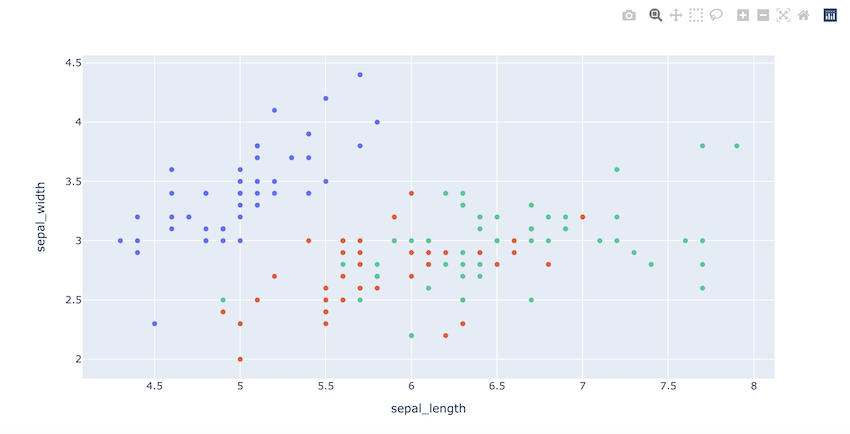By default showlegend Parameter is true. When we draw a plot in plotly legend is always displayed.

## Python3

 `# import packages ` `import` `plotly.express as px ` `import` `pandas as pd ` ` `  `# importing csv file ` `df ``=` `pd.read_csv(``"iris.csv"``) ` ` `  `# scatter plot using plotly ` `fig ``=` `px.scatter(df, x``=``"sepal_length"``,  ` `                 ``y``=``"sepal_width"``,  ` `                 ``color``=``"species"``) ` ` `  `fig.show() `

Output: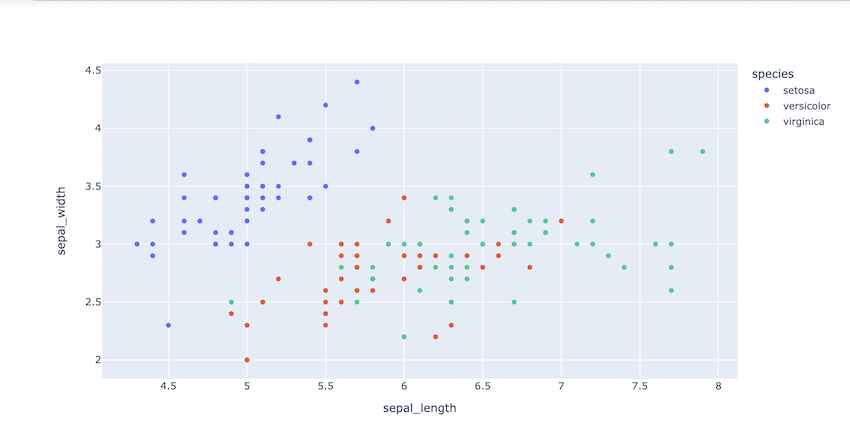### Example 2: Changing order of legend

In the below code we introduce a new parameter, legend_traceorder, and initialize is to “reversed”, the order of the legend is reversed by doing so.

## Python3

 `# import packages ` `import` `plotly.express as px ` `import` `pandas as pd ` ` `  `# importing csv file ` `df ``=` `pd.read_csv(``"iris.csv"``) ` ` `  `# scatter plot using plotly ` `fig ``=` `px.scatter(df, x``=``"sepal_length"``, ` `                 ``y``=``"sepal_width"``, ` `                 ``color``=``"species"``) ` ` `  `# order of legend is reversed ` `fig.update_layout(legend_traceorder``=``"reversed"``) ` ` `  `fig.show() `

Output:

Before changing order:After changing order: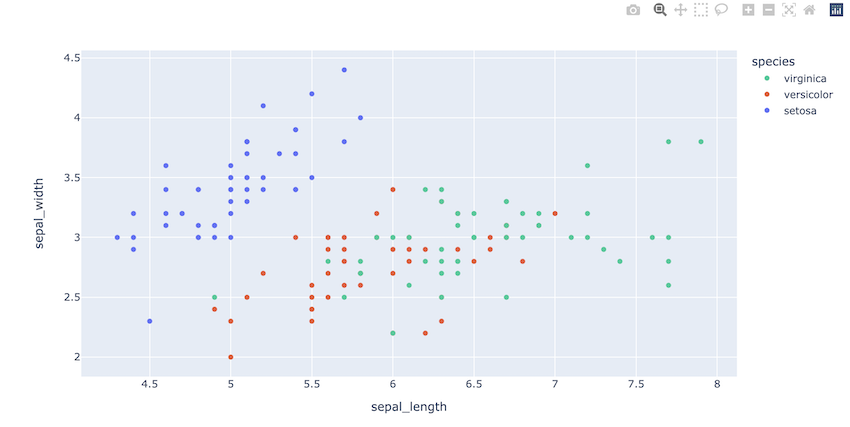The order setosa, versicolor , verginica is changed to virginica, versicolour , setosa.

### Example 3: Changing orientation of the legend

For a horizontal legend, set the layout.legend.orientation attribute to “h.” We also put it over the plotting area here. Generally, the legend is displayed vertically.

## Python3

 `# import packages ` `import` `plotly.express as px ` `import` `pandas as pd ` ` `  `# importing csv file ` `df ``=` `pd.read_csv(``"iris.csv"``) ` ` `  `# scatter plot using plotly ` `fig ``=` `px.scatter(df, x``=``"sepal_length"``, ` `                 ``y``=``"sepal_width"``,  ` `                 ``color``=``"species"``) ` ` `  `# changing orientation of the legend ` `fig.update_layout(legend``=``dict``( ` `    ``orientation``=``"h"``, ` ` `  `)) ` `fig.show() `

Output: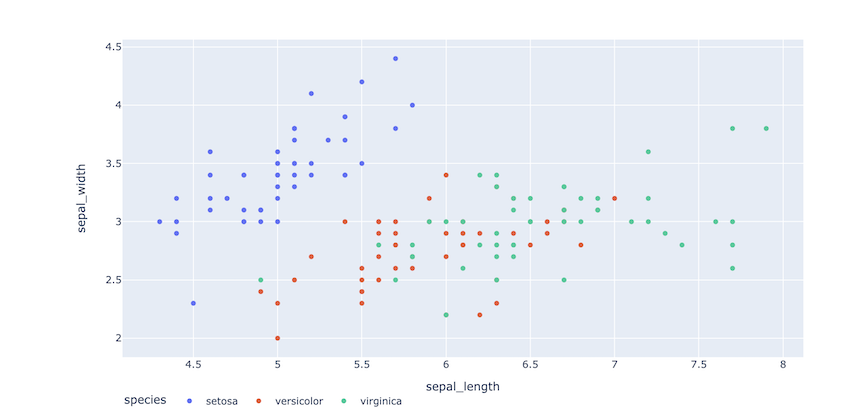### Example 4: Changing size, font, and color of legend

In this example, many other parameters are introduced, such as title_font_family, font where a dictionary of sub-parameters are specified for styling, bgcolor which is background colour, border colour and border width.

## Python3

 `# import packages ` `import` `plotly.express as px ` `import` `pandas as pd ` ` `  `# importing csv file ` `df ``=` `pd.read_csv(``"iris.csv"``) ` ` `  `# scatter plot using plotly ` `fig ``=` `px.scatter(df, x``=``"sepal_length"``, ` `                 ``y``=``"sepal_width"``, ` `                 ``color``=``"species"``) ` ` `  `# adding different style parameters to the legend ` `fig.update_layout( ` `    ``legend``=``dict``( ` `        ``x``=``0``, ` `        ``y``=``1``, ` `        ``title_font_family``=``"Times New Roman"``, ` `        ``font``=``dict``( ` `            ``family``=``"Courier"``, ` `            ``size``=``12``, ` `            ``color``=``"black"` `        ``), ` `        ``bgcolor``=``"LightBlue"``, ` `        ``bordercolor``=``"Black"``, ` `        ``borderwidth``=``1` `    ``) ` `) ` `fig.show() `

Output: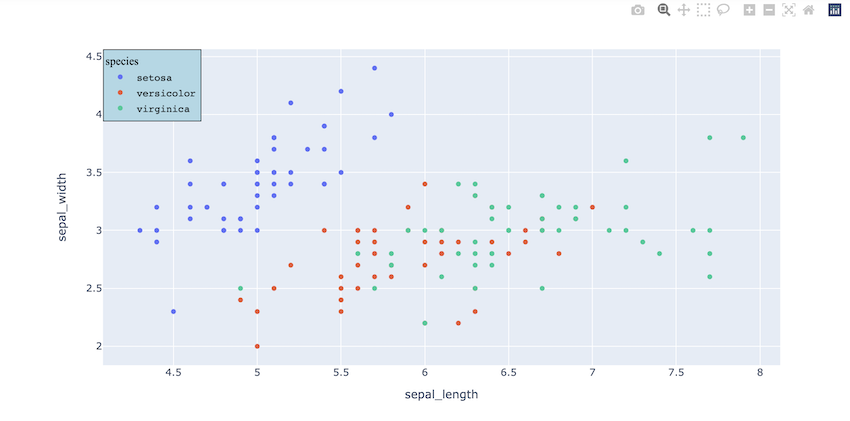My Personal Notes arrow_drop_up
Related Articles